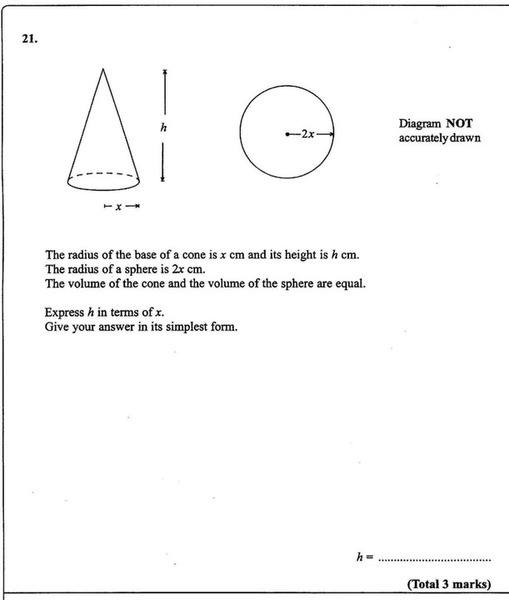# Expressing h in terms of x Cone/Sphere non-calc

• thomas49th

#### thomas49th

How do I do:the formula for volume of a cone is $$\frac{1}{3} \pi r^{2}h$$
and for sphere volume is $$\frac{4}{3} \pi r^{3}$$

It says express h in terms of x, so the equation will look somthing like

x =

Can someone give me help on how to rearrange this formula?
Thxequate the volumes and replace r with appropriate values

thomas49th said:
How do I do:

the formula for volume of a cone is $$\frac{1}{3} \pi r^{2}h$$
and for sphere volume is $$\frac{4}{3} \pi r^{3}$$

It says express h in terms of x, so the equation will look somthing like

x =

Can someone give me help on how to rearrange this formula?

You don't have an equation to rearrange yet! You need to express the volumes of the cone and sphere in terms of the variables you are given in this question. Note that "r" for the cone is not equal to "r" for the sphere in this case. What is each one equal to?

Once you have found this, you know that the volumes of the two objects are equal. This should enable you to obtain an equation.

$$v = \frac{1}{3} \pi x^{2}h$$

$$v = \frac{4}{3} \pi (2x)^{3}$$

Ok, now the volumes are the same, so can you combine these into one equation?

what u need to do now is to equal the two right sides, like doing v=v, just substitute the both expressions for v. then try to multiply by 3 and then divide by (pi*x^2) and you will get h in terms of x.

thomas49th said:
It says express h in terms of x, so the equation will look somthing like

x =

Thxand the equation will look something like

h=

$$\frac{4}{3} \pi x^{3}$$ = $$\frac{1}{3} \pi x^{2}h$$

$$\frac{4}{3} \pi 8x^{3}$$ = $$\frac{1}{3} \pi x^{2}h$$

divide by $$\frac{1}{3} \pi x^{2}$$

gives

$$h = 32x$$

is that right?

I did the math and that's what I got too.

yeah i guess that's right. good job

how come I can't edit these posts anymore... the edit button is gone. I can do it with other threads. I want to edit post 8, change $$\frac{4}{3} \pi x^{3}$$
to $$\frac{4}{3} \pi 2x^{3}$$

EDIT: I can edit post, but not my previous

thomas49th said:
how come I can't edit these posts anymore... the edit button is gone. I can do it with other threads. I want to edit post 8, change $$\frac{4}{3} \pi x^{3}$$
to $$\frac{4}{3} \pi 2x^{3}$$

EDIT: I can edit post, but not my previous

You can only edit upto 24 hours after posting.